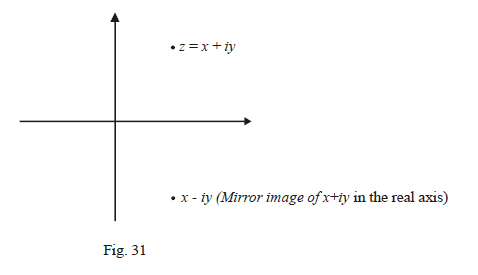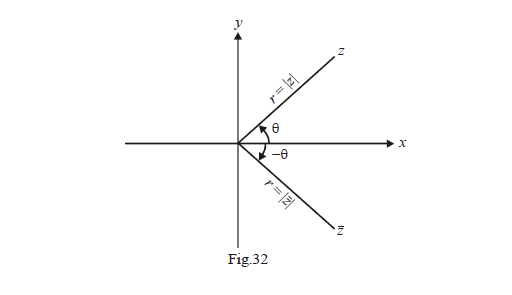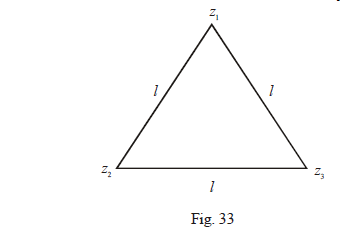# Conjugates Of Complex Numbers

Go back to  'Complex Numbers'

CONJUGATION:

Consider a complex number $$z = x + iy .$$ Where do you think will the number $$x - iy$$ lie? A little thinking will show that it will be the exact mirror image of the point $$z$$, in the x-axis mirror.Such a number is given a special name. It is called the conjugate of $$z$$ and represented as $$\bar z$$. Therefore,

if $$z = x + iy,$$ then  $$\bar z = x - iy$$ .

It turns out the notion of a conjugate is, though simple, very useful. We will put the conjugate to a lot of use in this chapter.

Let us now see some of the properties of the conjugate.

(1)  $$\left| z \right| = \left| {\bar z} \right| \text{ and } \arg (\bar z) = - \arg (z)$$ .

The validity of these two relations should be obvious from the figure below:(2) $$\overline {{z_1} \pm {z_2}} = {\bar z_1} \pm {\bar z_2}$$

These relations mean that the operation of conjugation is distributive over addition (and subtraction). You are urged to verify this by considering two arbitrary complex numbers $${z_1} = {x_1} + i{y_1}$$  and  $${z_2} = {x_2} + i{y_2}$$ .

(3)  \begin{align}\overline {{z_1}{z_2}} = {\bar z_1}{\bar z_2};\,\,\,\,\overline {\left( {\frac{{{z_1}}}{{{z_2}}}} \right)} = \frac{{{{\bar z}_1}}}{{{{\bar z}_2}}}\end{align}

These relations imply that conjugation is distributive over multiplication and division too. Lets prove this for multiplication.

Let $${z_1} = {x_1} + i{y_1}$$  and  $${z_2} = {x_2} + i{y_2}$$  .

Then,   $$\overline {{z_1}{z_2}} = \overline {({x_1} + i{y_1})({x_2} + i{y_2})}$$ .

$$= \overline {({x_1}{x_2} - {y_1}{y_2}) + i({x_1}{y_2} + {x_2}{y_1})}$$ .

$$= ({x_1}{x_2} - {y_1}{y_2}) - i({x_1}{y_2} + {x_2}{y_1})$$

Now,   $${\bar z_1}{\bar z_2} = \overline {({x_1} + i{y_1})} \,\,\,\overline {({x_2} + i{y_2})}$$ .

$$= ({x_1} - i{y_1})({x_2} - i{y_2})$$ .

$$= ({x_1}{x_2} - {y_1}{y_2}) - i({x_1}{y_2} + {x_2}{y_1})$$

We see that $$\overline {{z_1}{z_2}} = {\bar z_1}{\bar z_2}$$ . The proof for division is similar.

(4)  $${\left| z \right|^2} = z\bar z$$

This is one of the most important relations to be used in this chapter. The proof is very straightforward.

Let $$z = x + iy.$$

\begin{align}z\bar z &= (x + iy)(x - iy)\\&= {x^2} - {i^2}{y^2}\\&= {x^2} + {y^2}\\&= {(\sqrt {{x^2} + {y^2}} )^2}= {\left| z \right|^2}\end{align}

(5)  $$z + \bar z = 2{\mathop{\rm Re}\nolimits} (z);\,\,z - \bar z = 2i{\mathop{\rm Im}\nolimits} (z)$$ . $${\mathop{\rm Re}\nolimits} (z)$$  denotes the real part of z and Im(z) denotes the imaginary part of $$z.$$

This property is again obvious; just consider  $$z = x + iy$$  to deduce it.

Example- 6

Simply the following

(a)  $${\left| {{z_1} \pm {z_2}} \right|^2}$$                    (b)  $${\left| {{z_1} + {z_2}} \right|^2} + {\left| {z{{\kern 1pt} _1} - {z_2}} \right|^2}$$

Solution: (a) Let us simplify  $${\left| {{z_1} + {z_2}} \right|^2}$$

\begin{align}{\left| {{z_1} + {z_2}} \right|^2} &= ({z_1} + {z_2})(\overline {{z_1} + {z_2}} ) \qquad \qquad \text{(property 4)}\\&= ({z_1} + {z_2})({\bar z_1} + {\bar z_2}) \qquad \qquad \text{(property 2)}\\& = {z_1}{\bar z_1} + {z_2}{\bar z_2} + {z_1}{\bar z_2} + {\bar z_1}{z_2}\\&= {\left| {{z_1}} \right|^2} + {\left| {{z_2}} \right|^2} + {z_1}{\bar z_2} + {\bar z_1}{z_2}\end{align}

Now notice that $${\bar z_1}{z_2}$$  can be written as  $$\overline {{z_1}{{\bar z}_2}}$$ by virtue of property (3) $$\{ \overline {{z_1}{{\bar z}_2}} = {\bar z_1}{\overline{\overline {\,z\,}} _2} = {\bar z_1}{z_2}\}$$. Therefore,  $${z_1}{\bar z_2} + {\bar z_1}{z_2}$$  becomes  $${z_1}{\bar z_2} + \overline {{z_1}{{\bar z}_2}}$$ which is equal to $$2{\mathop{\rm Re}\nolimits} ({z_1}{\bar z_2})$$ by property (5).

Hence,

${\left| {{z_1} + {z_2}} \right|^2} = {\left| {{z_1}} \right|^2} + {\left| {{z_2}} \right|^2} + 2{\mathop{\rm Re}\nolimits} ({z_1}{\bar z_2})$

Similarly,

${\left| {{z_1} - {z_2}} \right|^2} = {\left| {{z_1}} \right|^2} + {\left| {{z_2}} \right|^2} - 2{\mathop{\rm Re}\nolimits} ({z_1}{\bar z_2})$

(b) The solution to this part is obtained by simply adding the two expressions obtained in part (a):

${\left| {{z_1} + {z_2}} \right|^2} + {\left| {{z_1} - {z_2}} \right|^2} = 2({\left| {{z_1}} \right|^2} + {\left| {{z_2}} \right|^2})$

Example- 7

If  $${z_1},\,\,{z_2},\,\,{z_3}\,$$ represent the vertices of an equilateral triangle, show that \begin{align}\frac{1}{{{z_1} - {z_2}}} + \frac{1}{{{z_2} - {z_3}}} + \frac{1}{{{z_3} - {z_1}}} = 0\end{align} .

Solution:We know that the sides of an equilateral triangle are equal. Using this for the triangle above, we get:

$\left| {{z_1} - {z_2}} \right| = \left| {{z_2} - {z_3}} \right| = \left| {{z_3} - {z_1}} \right| = l$

Now, we square this to get

${\left| {{z_1} - {z_2}} \right|^2} = {\left| {{z_2} - {z_3}} \right|^2} = {\left| {{z_3} - {z_1}} \right|^2} = {l^2}$

or $$({z_1} - {z_2})({\bar z_1} - {\bar z_2}) = ({z_2} - {z_3})({\bar z_2} - {\bar z_3}) = ({z_3} - {z_1})({\bar z_3} - {\bar z_1}) = {l^2}$$ (properties 5 and 2)

\begin{align}\Rightarrow \qquad \frac{1}{{{z_1} - {z_2}}} = \frac{{{{\bar z}_1} - {{\bar z}_2}}}{{{l^2}}};\,\,\,\,\,\frac{1}{{{z_2} - {z_3}}} = \frac{{{{\bar z}_2} - {{\bar z}_3}}}{{{l^2}}};\,\,\,\,\,\frac{1}{{{z_3} - {z_1}}} = \frac{{{{\bar z}_3} - {{\bar z}_1}}}{{{l^2}}}\end{align}

Adding these three, we get the desired result.

Example- 8

Prove that if the sum and product of two non-real complex numbers are real, they must be the conjugates of each other.

Solution: Let $${z_1} = {x_1} + i{y_1} \,\,\,\,\text{and} \,\,\,\,{z_2} = {x_2} + i{y_2} \,\,\,\,\,\,\,\,\text{where }{y_1},{y_2} \ne 0$$ .

It is given that   $${z_1} + {z_2}$$  and  $${z_1}{z_2}$$ are purely real, i.e.,

${\mathop{\rm Im}\nolimits} ({z_1} + {z_2}) = {\mathop{\rm Im}\nolimits} ({z_1}{z_2}) = 0$

\begin{align}&{\mathop{\rm Im}\nolimits} ({z_1} + {z_2}) = {\mathop{\rm Im}\nolimits} ({z_1}{z_2}) = 0\\&\Rightarrow {y_1} + {y_2} = 0\;\,\,\text{and} \;\;{x_1}{y_2} + {x_2}{y_1} = 0\\&\Rightarrow {y_1} = - {y_2}\,\,\;\text{and}\;\; {x_1}{y_2} - {x_2}{y_2} = 0\\& \Rightarrow {x_1} = {x_2}\end{align}

Thus, $${z_1} = {x_1} + i{y_1} \; and \; {z_2} = {x_2} + i{y_2} = {x_1} - i{y_1}$$  or in other words,

$${z_1} \; \text{and} \; {z_2}$$ are conjugates of each other.

Example- 9

If  $${\left| {{z_1} + {z_2}} \right|^2} = {\left| {{z_1}} \right|^2} + {\left| {{z_2}} \right|^2}$$  for two arbitrary non-zero complex numbers, show that \begin{align}\frac{{{z_1}}}{{{z_2}}}\end{align} is purely imaginary.

Solution:  From example-6,$${\left| {{z_1} + {z_2}} \right|^2}$$  can be written as  $${\left| {{z_1}} \right|^2} + {\left| {{z_2}} \right|^2} + 2{\mathop{\rm Re}\nolimits} ({z_1}{\bar z_2})$$

$$\Rightarrow$$  $${\left| {{z_1}} \right|^2} + {\left| {{z_2}} \right|^2} + 2{\mathop{\rm Re}\nolimits} \,({z_1}{\bar z_2}) = {\left| {{z_1}} \right|^2} + {\left| {{z_2}} \right|^2}$$

$$\Rightarrow$$ $${\mathop{\rm Re}\nolimits} ({z_1}{\bar z_2}) = 0$$

Since the real part of $${z_1}{\bar z_2}$$ is 0 , it must be purely imaginary. This means that it lies on the imaginary axis or its argument must be \begin{align}\frac{\pi }{2}{\rm{or}}\frac{{ - \pi }}{2}\end{align} .

\begin{align}&\Rightarrow \qquad Arg ({z_1}{\bar z_2}) = \pm \frac{\pi }{2}\\&\Rightarrow \qquad Arg ({z_1}) + Arg({\bar z_2}) = \pm \frac{\pi }{2} \qquad \text{[Arguments of complex number when they are multiplied]}\\ &\Rightarrow \qquad {\rm{Arg}}({z_1}) - {\rm{Arg}}({z_2}) = \pm \frac{\pi }{2} \qquad [Arg (\bar z) = -Arg(z)]\\&\Rightarrow \qquad Arg \left( {\frac{{{z_1}}}{{{z_2}}}} \right) = \pm \frac{\pi }{2} \qquad \qquad \;\;\; \text{[Argument of complex numbers subtract when they are divided]}\end{align}

This shows that  \begin{align}\frac{{{z_1}}}{{{z_2}}}\end{align}  lies on  the imaginary axis; therefore, it is purely imaginary.

Example- 10

If  $${z_1},{z_2}....{z_n}$$ are complex numbers and $$\left| {{z_1}} \right| = \left| {{z_2}} \right| = ... = \left| {{z_n}} \right| = 1,$$ show that

$\left| {{z_1} + {z_2} + ... + {z_n}} \right| = \left| {\frac{1}{{{z_1}}} + \frac{1}{{{z_2}}} + ... + \frac{1}{{{z_n}}}} \right|$

Solution: The information that all the moduli are 1 and the form of the expression we need to obtain hint that we should consider the term  $${\left| {{z_i}} \right|^2}$$  :

\begin{align}&{\left| {{z_i}} \right|^2} = {z_i}{\bar z_i} = 1\\&\Rightarrow {z_i} = \frac{1}{{{{\bar z}_i}}} = \overline {\left( {\frac{1}{{{z_i}}}} \right)}\\&\Rightarrow \sum\limits_{i = 1}^n \left[ {\left| z \right|} = {\left| {\bar z} \right|} \right]{{z_i}} = \sum\limits_{i = 1}^n {\overline {\left( {\frac{1}{{{z_i}}}} \right)} } \\&= \overline {\left( {\sum\limits_{i = 1}^n {\frac{1}{{{z_i}}}} } \right)} \qquad \quad \text{[Conjugation distributes over addition]}\\&\Rightarrow \left| {\sum\limits_{i = 1}^n {{z_i}} } \right| = \left| {\overline {\left( {\sum\limits_{i = 1}^n {\frac{1}{{{z_i}}}} } \right)} } \right|\\&= \left| {\left( {\sum\limits_{i = 1}^n {\frac{1}{{{z_i}}}} } \right)} \right|\end{align}

This is the result we needed to obtain.

Learn from the best math teachers and top your exams

• Live one on one classroom and doubt clearing
• Practice worksheets in and after class for conceptual clarity
• Personalized curriculum to keep up with school